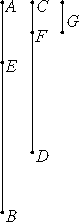# Proposition 2

To find the greatest common measure of two given numbers not relatively prime.

Let AB and CD be the two given numbers not relatively prime.

It is required to find the greatest common measure of AB and CD.If now CD measures AB, since it also measures itself, then CD is a common measure of CD and AB. And it is clear that it is also the greatest, for no greater number than CD measures CD.

But, if CD does not measure AB, then, when the less of the numbers AB and CD being continually subtracted from the greater, some number is left which measures the one before it.

For a unit is not left, otherwise AB and CD would be relatively prime, which is contrary to the hypothesis.

Therefore some number is left which measures the one before it.

Now let CD, measuring BE, leave EA less than itself, let EA, measuring DF, leave FC less than itself, and let CF measure AE.

Since then, CF measures AE, and AE measures DF, therefore CF also measures DF. But it measures itself, therefore it also measures the whole CD.

But CD measures BE, therefore CF also measures BE. And it also measures EA, therefore it measures the whole BA.

But it also measures CD, therefore CF measures AB and CD. Therefore CF is a common measure of AB and CD.

I say next that it is also the greatest.

If CF is not the greatest common measure of AB and CD, then some number G, which is greater than CF, measures the numbers AB and CD.

Now, since G measures CD, and CD measures BE, therefore G also measures BE. But it also measures the whole BA, therefore it measures the remainder AE.

But AE measures DF, therefore G also measures DF. And it measures the whole DC, therefore it also measures the remainder CF, that is, the greater measures the less, which is impossible.

Therefore no number which is greater than CF measures the numbers AB and CD. Therefore CF is the greatest common measure of AB and CD.

Q.E.D.

# Corollary

From this it is clear that, if a number measures two numbers, then it also measures their greatest common measure.

## Guide

Euclid again uses antenaresis (the Euclidean algorithm) in this proposition, this time to find the greatest common divisor of two numbers that aren’t relatively prime. Had Euclid considered the unit (1) to be a number, he could have merged these two propositions into one.

#### The Euclidean algorithm, antenaresis

The greatest common divisor of two numbers m and n is the largest number which divides both. It’s usually denoted GCD(m, n). It can be found using antenaresis by repeatedly subtracting the smaller, whichever it happens to be at the time, from the larger until the smaller divides the larger.

As an illustration consider the problem of computing the greatest common divisor of 884 and 3009. First, repeatedly subtract 884 from 3009 until the remainder is less than 884. An equivalent numerical operation is to divide 884 into 3009; you’ll get the same remainder. In this case after subtracting 884 three times, the remainder is 357. The two numbers under our consideration are now 884 and 357. Repeatedly subtract 357 from 884 to get the remainder 170. Repeatedly subtract 170 from 357 to get the remainder 17. Finally, stop since 17 divides 170. We’ found that GCD(884, 3009) equals 17.

The stages of the algorithm are the same as in VII.1 except that the final remainder an+1, which divides the previous number an, is not 1.

a1 – m1 a2 = a3
a2 – m2 a3 = a4
...
an-1 – mn-1 an = an+1.

(In Euclid’s proof a1 is AB, a2 is CD, a3 is AE, and a4 = an+1 is CF.)

In the first part of the proof, Euclid shows that since an+1 divides an, it also divides an-1, ... , a2, and a1. Therefore an+1 is a common divisor of a2 and a1. In the last part of the proof, Euclid shows that if any number d divides both a2 and a1, then it also divides a3, ... , an, and an+1. Therefore an+1 is the greatest common divisor. The last part of the proof also shows that every common divisor divides the greatest common divisor as noted in the corollary.

#### Foundations of number theory

Euclid makes many implicit assumptions about numbers. For instance, he assumes that if a < b, then a can be repeatedly subtracted from b until there is eventually a remainder less than or equal to a. He seems to have recognized that magnitudes need not have this property since the property is used as a qualifier in the definition of ratios (V.Def.4), but he didn’t recognize its importance for numbers. There are, in fact, nonstandard models of number theory which satisfy the usual properties of numbers, but do not have this property. In such models, there are numbers than can be decreased by 1 infinitely many times but not ever reach 1. The existence of such models implies an axiom is needed to exclude such behavior.

There is a similar assumption that the process of antenaresis eventually reaches an end when applied to numbers. Euclid certainly knew it needn’t halt for magnitudes since its halting is used as a criterion for incommensurability (X.2).

There needs to be an explicit axiom to cover these situations. One such axiom is a descending chain condition which states that there is no infinite decreasing sequence of numbers

a1 > a2 > ... > an > ...

#### Use of this proposition

This proposition and its corollary are used in the next two propositions.

Note how similar this proposition is to X.3, even having the same diagram and the same corollary. The terminology is slightly different and X.3 deals with magnitudes rather than numbers.